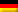Flow rate Inlet into the distillate receiver (approximate density of benzene used at 20 °C):

The container should be able to serve as a buffer for 2 hours until filling, i.e. it should hold at least a volume of approx.

V = qVD · t = 0,0003 m³/s · 7200 s = 2,2 m³

Selected (p. 167): Container with dished ends D = 1600 mm and L = 1.5 m (cylindrical part).

The filling volume is calculated according to the formula (p. 167):

According to the table on page 167, the volumes in the dished ends can be read off for the respective filling level. For example for a level h = 1000 mm VK = 0.55486 m³ applies

The support values in the table for the diagram V = f(h) are calculated accordingly:

h in mm

V in m³

200

0,25

400

0,72

600

1,29

800

1,91

1000

2,54

1200

3,11

1400

3,57

Dieser Beitrag ist auch verfügbar auf:Deutsch (German)